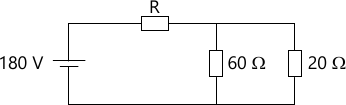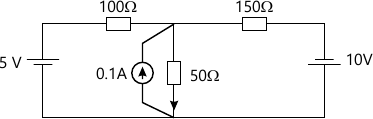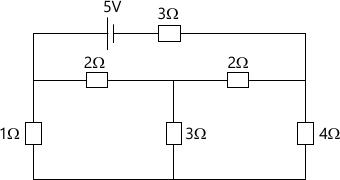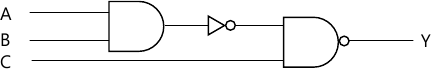MORE IN Basic Electricals & Electronics Engg.
RGPV First Year Engineering (Set A) (Semester 1)
Basic Electricals & Electronics Engg.
May 2012
Total marks: --
Total time: --
INSTRUCTIONS
(1) Assume appropriate data and state your reasons
(2) Marks are given to the right of every question
(3) Draw neat diagrams wherever necessary

Answer any Two of the following :
1 (a) State the NORTION'S Theorem. In the circuit shown below determine
(i) The value of R so that load of 20 ohm draws maximum power.
(ii) The value of maximum power drawn by the load.7 M
1 (b) State superposition theorem. Apply the same for finding the current in 5 ohm resister with the reference direction shown in circuit.7 M
1 (c) Determine the current drawn from the 5 volt battery in the network shown.7 M
1 (d) Explain the following terms :
(i) RMS
(ii) Average Value
(iii) Active Power
(iv) Reactive Power
7 M

Answer any Two of the following :
2 (a) Explain the following w.r.t. transformer (i) Losses (ii) Voltage regulation
7 M
2 (b) Draw the phasor diagram of single phase transformer with an individual load. Write down the procedure in steps for drawing the phasor diagram.
7 M
2 (c) A 11 KV/400 V distribution transformer takes a no load primary current of 1 Amp at a power factor of 0.24 lagging.
Find :
(i) The core loss current
(ii) The magnetizing current
(iii) The iron loss
7 M
2 (d) Give reasons why:
(i) Rating of transformer is specified in KVA and not in KW.
(ii) Core losses are called iron losses.
(iii) Cooling is required in transformer
(iv) Cores of transformer is laminated with laminated sheets
7 M

Answer any Two of the following :
3 (a) Draw torque-slip characteristics of a 3 phase inductions motor. Explain the concept of slip.
7 M
3 (b) Explain the principle of operation of transformer with suitable sketches.
7 M
3 (b) Give reasons why:
(i) Starting current is high in dc motor
(ii) Induced emf in a dc motor is called back emf
7 M
3 (c) Specify the application of following motor in field (minimum two)
(i) Three phase induction motor
(ii) Synchronous motor
(iii) DC motors
(iv) Single phase induction motor
7 M
3 (d) A 3 phase, 6 pole induction motor runs at 960 rmp on full load. It is supplied from a 4 pole alternator running at 1500 rpm. Calculate full load slip of the motor.
7 M

Answer any Two of the following :
4 (a) Obtain the following :
(i) Binary equivalent of (123.72)8
(ii) Octal equivalent of (10010110.1011)2
(iv) Decimal equivalent to (11011000)2
7 M
4 (b) Draw the truth table of the following logic circuit.7 M
4 (c) State and prove Demorgan's theorem using two variables.
7 M
4 (d) Explain the operations of following flip flops.
(i) J-K Flip Flop
(ii) R-S Flip Flop
7 M

Answer any Two of the following :
5 (a) Compare the CE, CB and CC configuration of BJT on the basis of -
(i) Input resistance
(ii) Output resistance
(iii) Voltage gain
(iv) Current gain
7 M
5 (b) Specify the following terms:
Forbidden energy gap
(ii) Intrinsic semiconductor
(iii) Doping
(iv) Charge carriers
(v) Biasing
7 M
5 (c) Explain the operation of BJT under following mode:
(i) Cut off mode
(ii) Active mode
(iii) Saturation mode
7 M
5 (d) Distinguish the following :
(i) Semiconductor and Insulator
(ii) P-Type and N-Type materials.
7 M

More question papers from Basic Electricals & Electronics Engg.